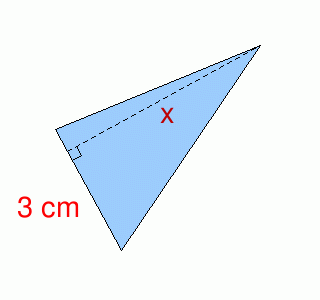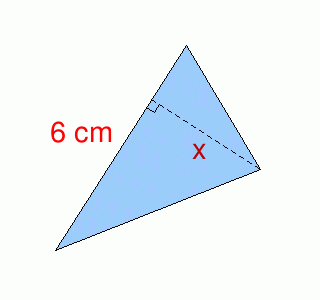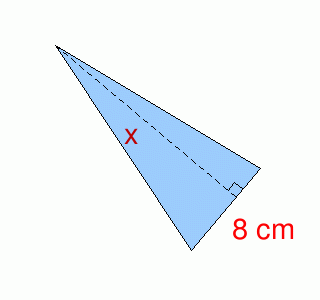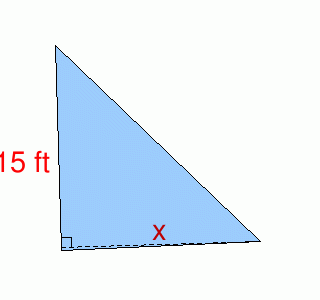MathScore EduFighter is one of the best math games on the Internet today. You can start playing for free!

## Triangle Area 2 - Sample Math Practice Problems

The math problems below can be generated by MathScore.com, a math practice program for schools and individual families. References to complexity and mode refer to the overall difficulty of the problems as they appear in the main program. In the main program, all problems are automatically graded and the difficulty adapts dynamically based on performance. Answers to these sample questions appear at the bottom of the page. This page does not grade your responses.

See some of our other supported math practice problems.

### Complexity=1, Mode=algebra

 1.   The area is 7.5 cm2.x = 2.   The area is 9 cm2.x =

### Complexity=1, Mode=word

 1.   What is the area of a triangle with a side length of 6ft and a height of 3ft? Area = 2.   What is the area of a triangle with a side length of 7ft and a height of 3ft? Area =

### Complexity=2, Mode=word

 1.   What is the area of a triangle with a side length of 14ft and a height of 19ft? Area = 2.   A triangle has an area of 60 yd2. The height is 8 yd. What is the length of the corresponding base? base =

### Complexity=3, Mode=algebra

 1.   The area is 68 cm2.x = 2.   The area is 112.5 ft2.x =

### Complexity=4, Mode=word

 1.   What is the area of a triangle with a side length of 18yd and a height of 50yd? Area = 2.   A triangle has an area of 324 in2. The length of one side is 36 in. What is the corresponding height? height =

### Complexity=1, Mode=algebra

1The area is 7.5 cm2.x =
Solution
A=1/2bh (one half base times height)
7.5 cm2 = 1/2(3cm * x)
15 cm2 = 3cm * x
5 cm = x
2The area is 9 cm2.x =
Solution
A=1/2bh (one half base times height)
9 cm2 = 1/2(6cm * x)
18 cm2 = 6cm * x
3 cm = x

### Complexity=1, Mode=word

1What is the area of a triangle with a side length of 6ft and a height of 3ft?
Area =
Solution
A=1/2bh (one half base times height)
A = 1/2(6ft * 3ft
A = 9 ft2
2What is the area of a triangle with a side length of 7ft and a height of 3ft?
Area =
Solution
A=1/2bh (one half base times height)
A = 1/2(7ft * 3ft
A = 10.5 ft2

### Complexity=2, Mode=word

1What is the area of a triangle with a side length of 14ft and a height of 19ft?
Area =
Solution
A=1/2bh (one half base times height)
A = 1/2(14ft * 19ft
A = 133 ft2
2A triangle has an area of 60 yd2. The height is 8 yd. What is the length of the corresponding base?
base =
Solution
A=1/2bh (one half base times height)
60 yd2 = 1/2(b * 8yd)
120 yd2 = b * 8yd
15 yd = b

### Complexity=3, Mode=algebra

1The area is 68 cm2.x =
Solution
A=1/2bh (one half base times height)
68 cm2 = 1/2(8cm * x)
136 cm2 = 8cm * x
17 cm = x
2The area is 112.5 ft2.x =
Solution
A=1/2bh (one half base times height)
112.5 ft2 = 1/2(15ft * x)
225 ft2 = 15ft * x
15 ft = x

### Complexity=4, Mode=word

1What is the area of a triangle with a side length of 18yd and a height of 50yd?
Area =
Solution
A=1/2bh (one half base times height)
A = 1/2(18yd * 50yd
A = 450 yd2